JMSLTM Numerical Library 7.2.0
com.imsl.stat

## Class FaureSequence

• All Implemented Interfaces:
RandomSequence, Serializable, Cloneable

```public class FaureSequence
extends Object
implements Serializable, RandomSequence, Cloneable```
Generates the low-discrepancy Faure sequence.

Discrepancy measures the deviation from uniformity of a point set.

The discrepancy of the point set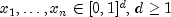, is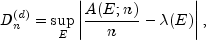where the supremum is over all subsets of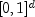of the form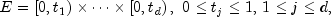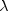is the Lebesque measure, and A(E;n) is the number of the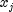contained in E.

The sequence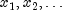of points inis a low-discrepancy sequence if there exists a constant c(d), depending only on d, such that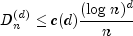for all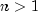.

Generalized Faure sequences can be defined for any prime base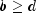. The lowest bound for the discrepancy is obtained for the smallest prime, so the base defaults to the smallest prime greater than or equal to the dimension.

The generalized Faure sequence, is computed as follows:

Write the positive integer n in its b-ary expansion,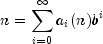where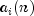are integers,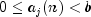.

The j-th coordinate of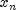is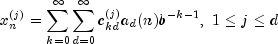The generator matrix for the series,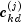, is defined to be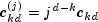and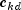is an element of the Pascal matrix,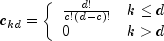It is faster to compute a shuffled Faure sequence than to compute the Faure sequence itself. It can be shown that this shuffling preserves the low-discrepancy property.

The shuffling used is the b-ary Gray code. The function G(n) maps the positive integer n into the integer given by its b-ary expansion. The sequence computed by this function is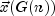, where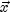is the generalized Faure sequence.

Example, Serialized Form
• ### Constructor Summary

Constructors
Constructor and Description
`FaureSequence(int dim)`
Creates a Faure sequence with the default base.
```FaureSequence(int dim, int base, int nSkip)```
Creates a Faure sequence.
• ### Method Summary

Methods
Modifier and Type Method and Description
`Object` `clone()`
Returns a copy of this object.
`int` `getBase()`
Returns the base.
`long` `getCount()`
`int` `getDimension()`
Returns the dimension of the sequence.
`int` `getSkip()`
Returns the number of points skipped at the beginning of the sequence.
`double` `nextDouble()`
Returns the first value of the next point in the sequence.
`double[]` `nextPoint()`
Returns the next point in the sequence.
`static int` `nextPrime(int n)`
Returns the smallest prime greater than or equal to n.
• ### Methods inherited from class java.lang.Object

`equals, finalize, getClass, hashCode, notify, notifyAll, toString, wait, wait, wait`
• ### Constructor Detail

• #### FaureSequence

`public FaureSequence(int dim)`
Creates a Faure sequence with the default base. The base defaults to the smallest prime equal to or greater than dim.
Parameters:
`dim` - is the dimension of the sequence.
• #### FaureSequence

```public FaureSequence(int dim,
int base,
int nSkip)```
Creates a Faure sequence.
Parameters:
`dim` - is the dimension of the sequence.
`base` - is the base of the sequence, as described above. It must be at least as large as dim.
`nSkip` - is the number of initial points to skip. If negative then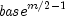, where m is the number of digits needed to represent the `Integer.MAX_VALUE` in the base, points are skipped.
• ### Method Detail

• #### clone

`public Object clone()`
Returns a copy of this object.
Overrides:
`clone` in class `Object`
• #### getBase

`public int getBase()`
Returns the base.
• #### getCount

`public long getCount()`
• #### getDimension

`public int getDimension()`
Returns the dimension of the sequence.
Specified by:
`getDimension` in interface `RandomSequence`
• #### getSkip

`public int getSkip()`
Returns the number of points skipped at the beginning of the sequence.
• #### nextDouble

`public double nextDouble()`
Returns the first value of the next point in the sequence. This method is intended for use when dim is 1.
Returns:
a `double` array, the next sequence value.
• #### nextPoint

`public double[] nextPoint()`
Returns the next point in the sequence.
Specified by:
`nextPoint` in interface `RandomSequence`
Returns:
a `double` array, the next point in the sequence.
• #### nextPrime

`public static int nextPrime(int n)`
Returns the smallest prime greater than or equal to n.
Parameters:
`n` - is the first number to try as a prime.
Returns:
a prime greater than or equal to n. If n is less than or equal to 2 then 2 is returned.
JMSLTM Numerical Library 7.2.0